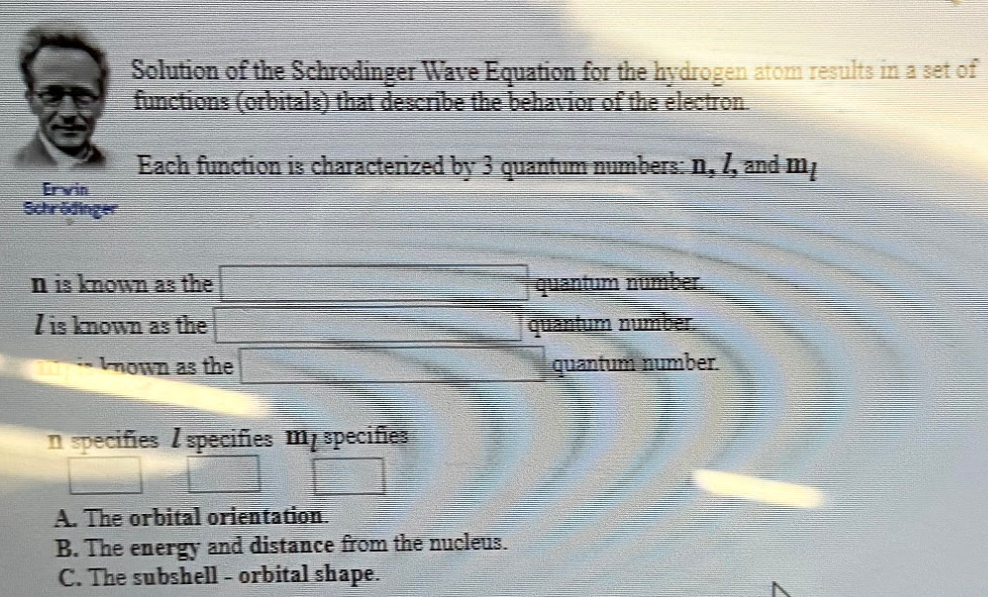# Solution of the Schrodinger Wave Equation for the hydrogen atom results in a set of functions (orbitals) that describe the behavior electron. Each function is characterized by 3 quantum numbers: n, l, and ml n is known as the ________ quantum number. l is known as the _________ quantum number . ml is known as the _________ quantum number. n specifies l specifies ml specifies A. The orbital orientation. B. The energy and distance from the nucleus. C. The subshell - orbital shape.